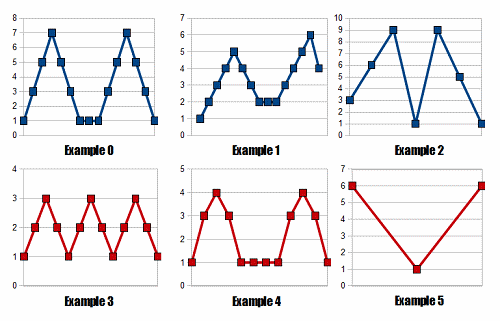### Problem Statement

Fox Ciel likes sequences. One day, she invented a new type of sequence and named it the fox sequence. A sequence seq containing N elements is called a fox sequence if and only if there exist four integers a, b, c and d such that 0 < a < b <= c < d < N-1 and the following five conditions are met:
• seq, seq, ... , seq[a] forms an arithmetic progression with a positive common difference. An arithmetic progression is a sequence where the difference between successive elements is equal. The difference between successive elements is called the common difference. Note that 0 is neither positive nor negative.
• seq[a], seq[a+1], ... , seq[b] forms an arithmetic progression with a negative common difference.
• seq[b], seq[b+1], ... , seq[c] are all equal.
• seq[c], seq[c+1], ... , seq[d] forms an arithmetic progression with a positive common difference.
• seq[d], seq[d+1], ... , seq[N-1] forms an arithmetic progression with a negative common difference.

In the following image, the top 3 sequences are fox sequences, while the bottom 3 sequences are not:You are given a sequence seq. Return "YES" if it is a fox sequence, or "NO" if it is not (all quotes for clarity).

### Definition

 Class: FoxSequence Method: isValid Parameters: int[] Returns: String Method signature: String isValid(int[] seq) (be sure your method is public)

### Constraints

-seq will contain between 1 and 50 elements, inclusive.
-Each element of seq will be between 1 and 2,000, inclusive.

### Examples

0)

 ```{1,3,5,7,5,3,1,1,1,3,5,7,5,3,1} ```
`Returns: "YES"`
 This is the top-left sequence of the image shown in the statement. The next five examples are also from that image.
1)

 ```{1,2,3,4,5,4,3,2,2,2,3,4,5,6,4} ```
`Returns: "YES"`
2)

 ```{3,6,9,1,9,5,1} ```
`Returns: "YES"`
3)

 ```{1,2,3,2,1,2,3,2,1,2,3,2,1} ```
`Returns: "NO"`
4)

 ```{1,3,4,3,1,1,1,1,3,4,3,1} ```
`Returns: "NO"`
5)

 ```{6,1,6} ```
`Returns: "NO"`

#### Problem url:

http://www.topcoder.com/stat?c=problem_statement&pm=11219

#### Problem stats url:

http://www.topcoder.com/tc?module=ProblemDetail&rd=14427&pm=11219

ir5

#### Testers:

PabloGilberto , ivan_metelsky , pieguy

#### Problem categories:

Simple Search, Iteration